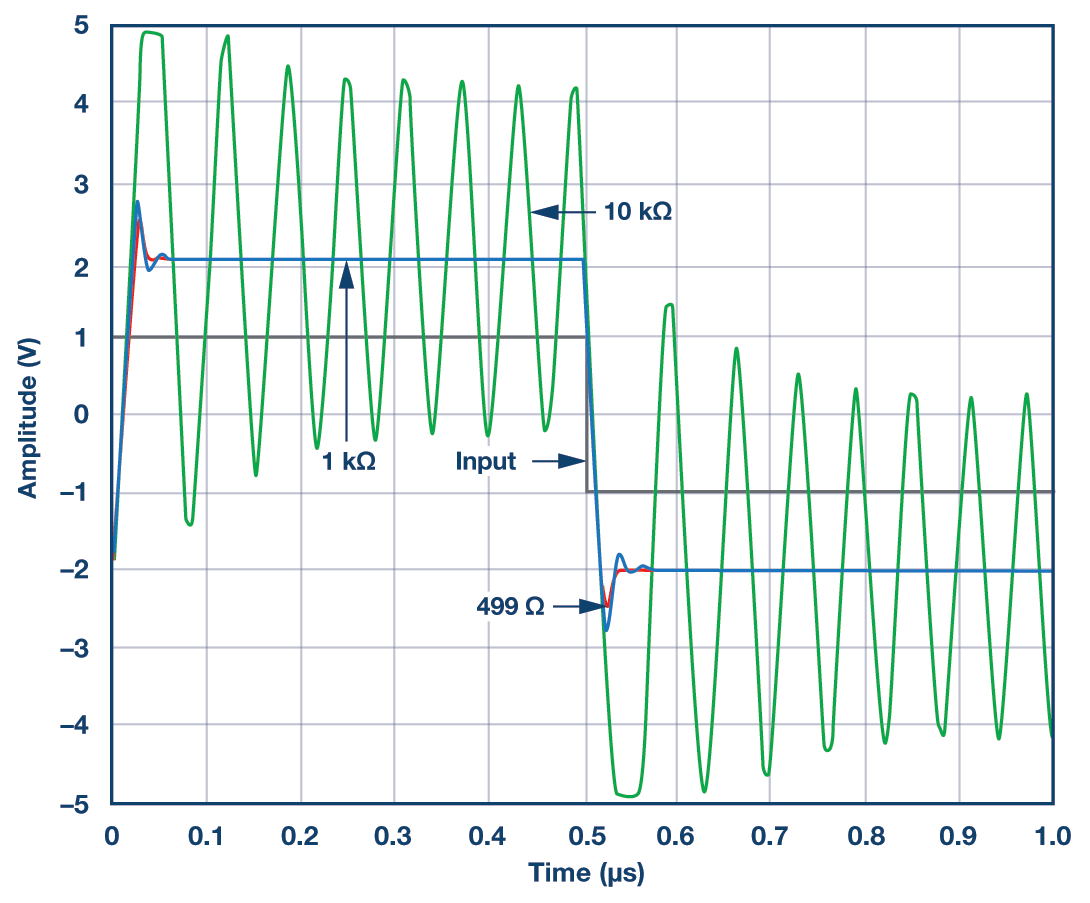# The Truth About Voltage Feedback Resistors

### QUESTION:

The stability of my fully differential and voltage feedback amplifiers seems highly impacted by the value of my feedback resistors—the RF/RG ratio is always correct, so what’s happening?

When a signal needs gain, an amplifier is the component of choice. The ratio of the feedback and gain resistors, RF/RG, for a voltage feedback and a fully differential amplifier, determines the gain. Once the ratio is set, the next step is to select a value for either RF or RG. The choice of RF can impact the stability of the amplifier.

An amplifier’s internal input capacitance, found in the specification table of the data sheet, interacts with RF to form a pole in the transfer function. If RF is exceedingly large, this pole will affect stability. If the pole occurs at a frequency much larger than the crossover frequency, it will not affect stability. However, if the location of the pole as determined by f = 1/(2πRFCin,amp) occurs near the crossover frequency, the phase margin will be reduced leading to potential instability.

The example of Figure 1 shows the lab results of the small signal closed-loop gain vs. frequency response for the ADA4807-1 voltage feedback amplifier in a noninverting gain of 2 configuration with feedback resistors 499 Ω, 1 kΩ, and 10 kΩ. The data sheet recommended RF value is 499 Ω.

The degree of peaking in the small signal frequency response indicates instability. Increasing RF from 499 Ω to 1 kΩ marginally increases peaking. This would imply the amplifier has sufficient phase margin with an RF of 1 kΩ and is stable. This is not the case for the RF of 10 kΩ. The high level of peaking present implies instability (oscillation) and it is not recommended.Figure 1. Lab results using different feedback resistors. VS = ±5 V, VOUT = 40 mV p-p, RLOAD = 1 kΩ for RF values of 499 Ω, 1 kΩ, and 10 kΩ.Figure 2. Simulation results using the ADA4807 SPICE model. VS = ±5 V, G = 2 and RLOAD = 1 kΩ for RF values of 499 Ω, 1 kΩ, and 10 kΩ.

Validating a circuit in the lab is not a mandatory step for verifying potential instabilities. Figure 3 shows the simulation results using the SPICE model with the same RF values of 499 Ω, 1 kΩ, and 10 kΩ. The results are consistent with Figure 1. Figure 3 shows the instability in the time domain. Adding a zero to the transfer function by placing a feedback capacitor across RF will remove the instability as shown in Figure 4.Figure 3. Pulse response simulation results using the ADA4807 SPICE model. VS = ±5 V, G = 2 and RLOAD = 1 kΩ for RF values of 499 Ω, 1 kΩ, and 10 kΩFigure 4. Pulse response simulation results with a 3.3 pF feedback capacitor, CF. VS = ±5 V, G = 2, RF = 10 kΩ and RLOAD = 1 kΩ.

There are trade-offs in the selection of RF, which are power dissipation, bandwidth, and stability. If power dissipation is critical, and the data sheet recommended feedback value cannot be used or a much higher RF value is necessary, placing a feedback capacitor in parallel with RF is an option. This choice results in lower bandwidth.

When selecting the RF for a voltage feedback and a fully differential amplifier, consideration needs to be given to the system requirements. If speed is not critical, a feedback capacitor will help to stabilize a large RF value. If speed is critical, the recommended data sheet RF value is advised. Ignoring the relationship of RF with respect to stability, bandwidth, and power, can hinder a system or, worse yet, prevent the system from achieving its full performance.